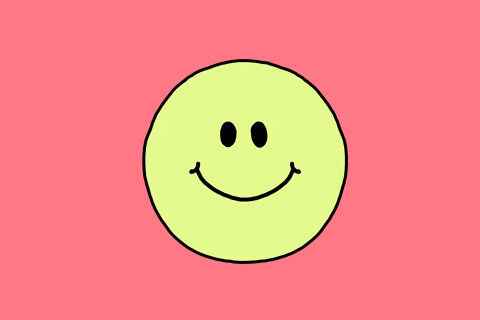#Comp 101 While Loop Examples

## While Loop Examples

With while loops, we can revisit a block of code again and again. Instead of copying and pasting code when we want something to repeat, we can simply place it within a while loop, and repeat as many times as we want!

...but if we don't have a good test for our while loop, it will go on forever.

``````let x = 0;
let y = 5;
while (0 < 5) {
print("Yay! " + x + " is less than " + y);
x = x + 1;
}``````

What will happen if we run the above code? It will run forever because the test in the while loop (is 0 < 5) will always be true. Regardless of the code within the block, if the condition in the loop begets infinite looping it will continue to loop.

``````let a = true;
let b = !a;
let c = false;

while (a) {
print("Hello!");
if (c) {
c = !c;
} else {
a = false;
}
}

*``````

Let's take a look at this code line by line and dissect what's happening. First, we initialize three variables; a is true, b is the opposite of a (which evaluates to be false), and c is false. Then, we have a while loop that continues to loop when a is true. Since we know a was initialized to be true, we enter the loop, and Hello! is printed. Since c is false, we skip ahead to the else block within the loop and assign a to be false.

Now that we've reached the end of our loop, we go up to the while test and ask if it evaluates to true. Is a true? No! Since we reassigned its value to be false at the end of the first loop, we exit the loop and skip ahead to where the asterisk in the code is.

Let's look at another code snippet:

``````let p = true;
let q  = false;
let r = (p || q);

if (p) {
while (q || r) {
p = false;
print(":-)");
}
}``````

We initialize three variables again, with p as true, q as false, and r as the result of evaluating p || q. Since true || false evaluates to be true, r is true. Now, arriving at an if-statement, we check if p is true. Since it is, we enter into the then-block.

Is q || r true? Since false || true is true, we enter into the if-block. p is now assigned to be false, and :-) is printed.

One common mistake is thinking that now that p is false, we must no longer continue in our while loop since the if-statement leading us to it is now no longer true. However, in a while loop we only look at the test for the while loop that we're in when determining whether or not to loop again. If it's true, we loop again. Else, we don't. It's that simple!

The result? That :-) is printed infinitely, because q || r evaluates to true for the rest of time!One last thing...

``````let i = 0;

while (i < 5) {
let j = 1;
while( j <= 3 ) {
print(j + " ");
j++;
}
print (":) ");
i++;
}``````

woaaaah check this out -- loop-ception!

We can have loops within our loops! Let's walk through this example together. We first initialize a variable i then construct a loop within which we initialize a variable j and construct another loop. Our outer loop uses i as a counter variable and runs while i is less than 5, while our inner loop uses j as a counter variable and runs while j is less than or equal to 3. Take a minute and think about what would be printed by this loop.

Did you get "1 2 3 :) 1 2 3 :) 1 2 3 :) 1 2 3 :) 1 2 3 :) "?

Notice that the inner loop starts its cycle over each time that the outer loop runs. This is because we declared our counter variable j within our outer loop, causing j to reset to 1 every time the outer loop runs.